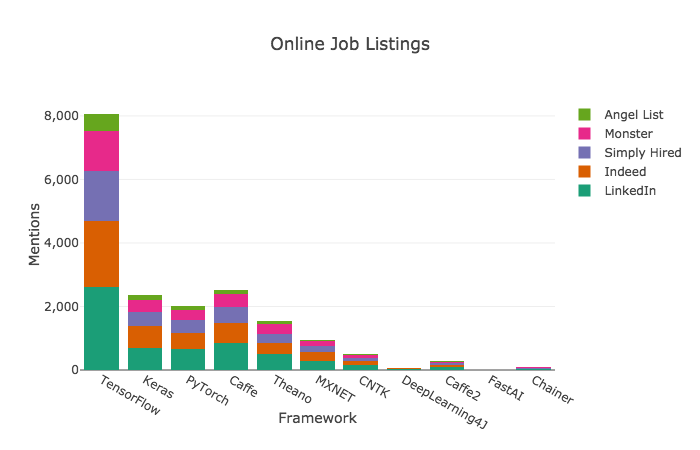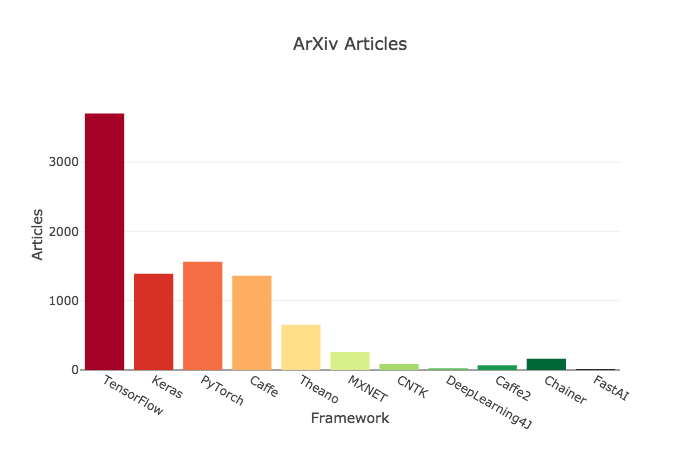#0

## 前言

TensorFlow 在去年就不斷的promote v2.0 版，到底它改善的方向有那些呢? 正式版何時release? 整理一些馬路新聞與大家分享。

## 市占率## 現況比較

PyTorch 攻擊 TensorFlow 論點是『TensorFlow 把簡單的問題複雜化，而 Keras 把複雜的問題太過簡單化』，最明顯的例子就是要建立一個『a+b』的運算圖，TensorFlow 程式如下：

``````import tensorflow as tf
import numpy as np
# Defining some constant values
a = tf.constant(5.0, name="a")
b = tf.constant(10.0, name="b")

# Some basic operations
with tf.Session() as sess:
print("x: ", sess.run(x))

# Closing the writer.
writer.close()
sess.close()
``````

## 重大更新

1. Eager execution：不需要建立 session，可直接使用 Python 語法建立模型，TensorFlow 會直接將IF等語法轉為運算圖，也可以在過程中除錯。
2. 依據 Keras 規格，改寫 Keras 套件功能，直接納入 TensorFlow 家族，它與獨立Keras套件的比較，請參看『Standardizing on Keras: Guidance on High-level APIs in TensorFlow 2.0』，主要強調Keras可與其他API整合(混用)及納入Eager execution功能。

``````import tensorflow as tf
import numpy as np
# 啟動 eager execution 模式
tf.enable_eager_execution()
a = tf.constant([[1, 2],
[3, 4]])
print(a+b)
``````

1. TensorFlow Serving 模組：可輕易的佈署在 HTTP 或 gRPC 協定上，提供線上服務。
2. TensorFlow Lite 模組：加強邊緣運算的支援，可佈署在手機、Raspberry Pi、Edge TPU 等。
3. TensorFlow.js 模組：提供 Node.js/JavaScript 開發，包括使用類似Keras的API。

## 結語

TensorFlow 的家族龐大，提供高低階的API，而且，模組替換很快，有些模組未來就不支援了，在學習時要注意，不要在行將就木的功能上鑽研，以免白忙一場。